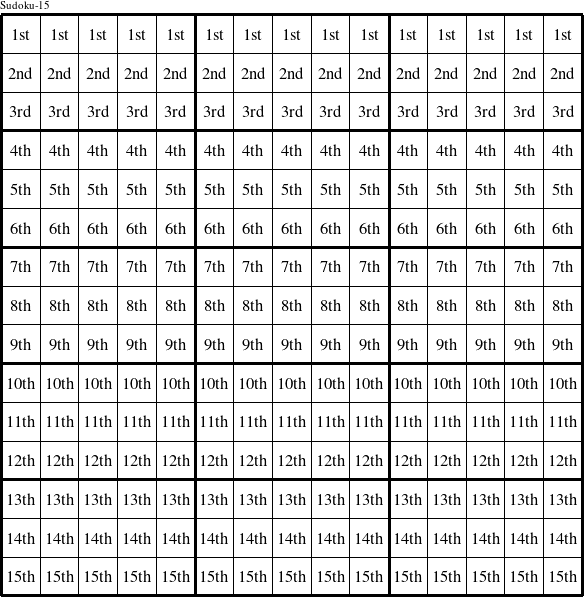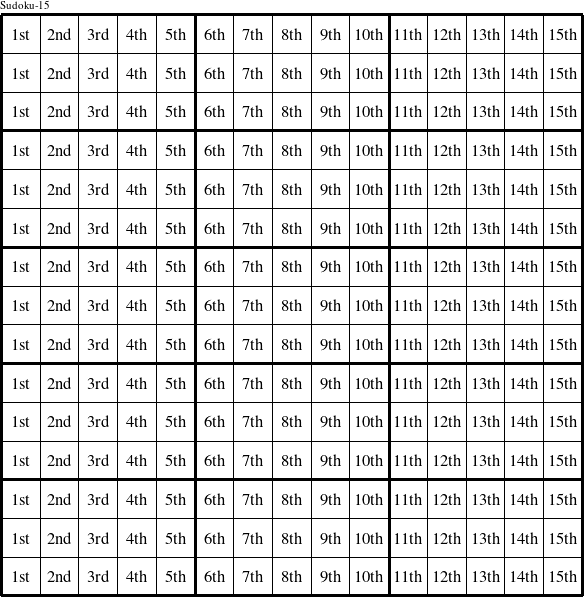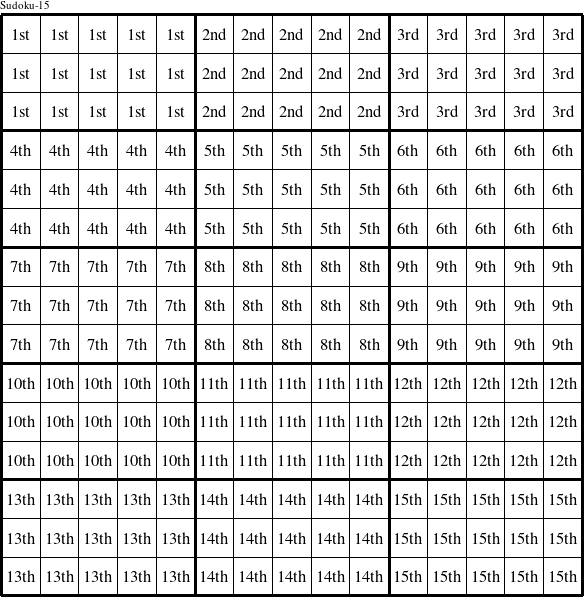#### Printable version

Detailed instructions for working a group puzzle of type 'Sudoku-15'

The 15 elements in each group of this type of puzzle are: 0, 1, 2, 3, 4, 5, 6, 7, 8, 9, A, B, C, D, and E.

Every group in the puzzle must contain exactly this set of elements.

####In a Sudoku-15 puzzle, each row is a group numbered as shown in the figure above.

####In a Sudoku-15 puzzle, each column is a group numbered as shown in the figure above.

####In a Sudoku-15 puzzle, each 5x3 rectangle is a group numbered as shown in the figure above.